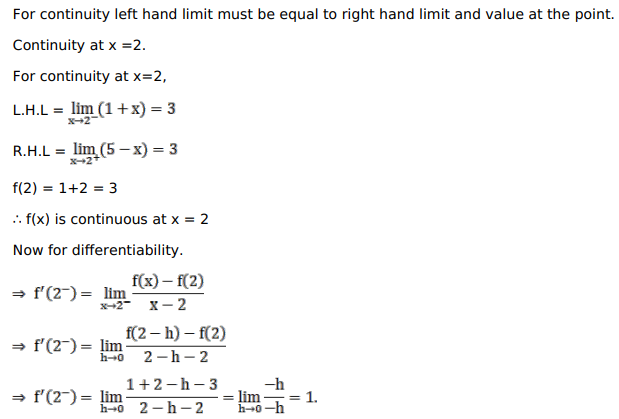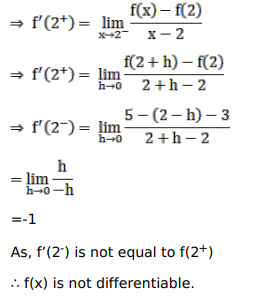# Solve this following

Question:

Mark $(\sqrt{)}$ against the correct answer in the following:

The function $f(x)=\left\{\begin{array}{ll}1+x, & \text { when } x \leq 2 \\ 5-x, & \text { when } x>2\end{array}\right.$ is

A. continuous as well as differentiable at $x=2$

B. continuous but not differentiable at $x=2$

C. differentiable but not continuous at $x=2$

D. none of these

Solution: